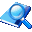万捷网络验证系统在线帮助文档
Help > WebApi接口说明 >

### 接口原型：

接口名称  说明
参数  说明

1=剩余秒数

2=剩余点数

3=允许几开

4=游戏号

5=上次登陆ip

6=邮箱

7=上次登录机器码

8=上次登录时间

9=用户权限

10=验证码(用于取回附加字符的必须参数)

11=到期时间

12=用户备注

13=注册时间。

### 访问示例：

http://服务器地址/api.php?name=接口名称&c1=软件编号&c2=账号&c3=密码&c4=软件版本&c5=机器码&c6=绑定信息&c7=1,2,3,4,5,6

### 在WebApi接口中的调用方法（将下面的代码复制到后台的WebApi代码框中）：

 function  zdy_ApiName(\$rjbh,\$zh,\$mm,\$bb,\$jqm,\$yxh,\$xxz){//请将 ApiName改为您自己想要定义的任意名称 \$data=login(\$rjbh,\$zh,\$mm,\$bb,\$jqm,\$yxh,\$xxz); return \$data; }